Open in App
Not now

# Marshallian Approach to Price Determination

• Last Updated : 12 Oct, 2022

Pursuing Economics as a subject or learning Economics as your interest, we all come across with the famous name ‘Alfred Marshall’. He was a British economist who shone in the late 1800s after the publication of his book “Principle of Economics”. This era was also known as the Marshallian era where he talked about many approaches and theories, where one approach was about price determination.

Before we delve into the Marshallian approach to price determination, we will first understand two approaches already existing about price determination.

1- Jewel utility theory of pricing

Jewel theory states that the price and utility are directly proportional to each other. Utility means the unit at which your level of satisfaction is measured.

2- Ricardian cost theory of pricing

Ricardian cost theory states that price and cost are directly proportional to each other.

Now let’s directly jump into our topic that is ‘Marshallian approach to price determination’

Marshall’s approach to price determination assumes that :

• The type of market is considered to be a perfect competition market.
• The terms supply and cost are the same.
• The terms demand and utility are the same.

Now, if we talk about price determination then the two factors that help in determining price are the forces of demand and supply. The demand and supply curve interaction helps in determining the equilibrium price in respect of quantity which means that demand factors or supply factors are solely not responsible for determining the equilibrium price.

As you can see in fig 1.1, the demand curve (downward sloping) and supply curve (upward sloping) both are responsible for determining the equilibrium price at the point of interaction. Point E is the equilibrium point (interaction point).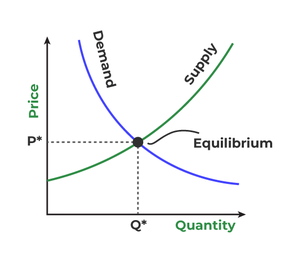According to Marshall, the supply curve is categorized into three types:

1-Downward sloping
2-Horizontal
3-Upward sloping

This depends on the laws of cost in the market. We will read about it thoroughly in different types of markets below.

### Types of Market :

There are three types of markets discussed in the Marshallian approach to price determination.

#### 1- Market period :

This is a very short run period. Herein, the only responsible variable is demand for determining equilibrium price because the supply is constant even if the price changes. Demand keeps on changing with the change in the price and a new equilibrium is formed.

As you can see in fig 1.2, the straight line is the supply curve as it is constant in the Market period. The demand curve (downward sloping) keeps on fluctuating with changes in price. E is the equilibrium price and shifts to E1 when the price increases, that is the new equilibrium price. Likewise, the equilibrium price shifts to E2 when the price falls.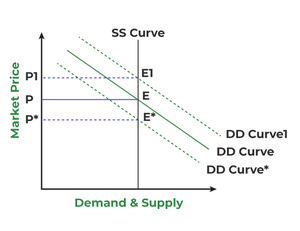Conclusion- Market prices and demand are directly proportional to each other where supply is constant in the Market period.

#### 2- Normal period :

The normal period is further divided into two categories:

1-Short run normal period
2-Long run normal period

Short-run Normal Period :

In this type of period the supply changes in a small amount with a change in price. The supply and demand factors are equally responsible for determining equilibrium prices.
In fig 1.3, we can determine that due to an increase in demand, the supply will increase, and due to an increase in supply, the price will also increase and vice versa. This denotes that supply and demand are directly proportional to price in the short run. The supply curve will be upward-sloping.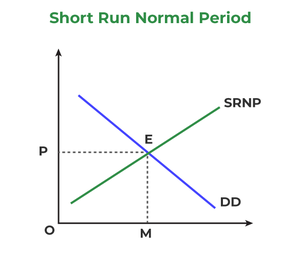Long-run Normal Period :

In this type of period the supply changes in adequate amounts with a change in price. The supply and demand factors are equally responsible for determining equilibrium prices. In fig 1.4, there is an increase in demand which leads to an increase in supply but a decrease in price and vice versa. The cause behind this is diminishing marginal utility. It denotes that demand and supply are inversely proportional to price.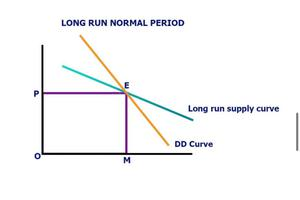Fig 1.4

Earlier we stated about laws of cost, let us read about types of ‘laws of cost‘ in the market. There are three types of laws of cost under long run normal period:

1- Law of constant cost
2- Law of increasing cost
3- Law of diminishing cost

#### Law of constant cost :

The law of constant cost states that the cost remains constant with the change in demand. In fig 1.5, we can see that cost(supply) is a horizontal line and the demand curve is downward sloping which determines the equilibrium price. So with an increase in demand, supply also increases and vice versa but price remains constant.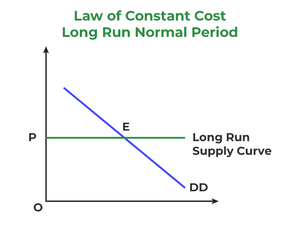#### Law of increasing cost :

Law of increasing cost states that there is an increase in cost which leads to an increase in price with respect to an increase in demand. In fig 1.6, we can clearly see that the cost(supply) curve is upward sloping and the demand curve is downward sloping which determines our equilibrium price. Everytime with an increase in demand, the price also increases.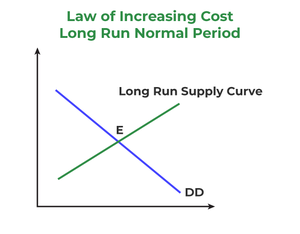#### Law of diminishing cost :

Law of diminishing cost states that there is a decrease in cost. The producer needs to increase production to come to an equilibrium point (MR = MC).

### 3- Secular period :

It is a very very long period where there is no certainty of any product whether its price over time is constant or increasing or decreasing. This time is considered to be very long, like a century.

For example, the necessity goods (salt) prices are constant over the years.

My Personal Notes arrow_drop_up
Related Articles